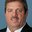Question-and-Answer Resource for the Building Energy Modeling Community
Get s tarted with the Help page

# Inputting Temperature Driftpoints in EnergyPlusI'm working on a simulation in EnergyPlus and I'm trying to figure out how to set drift points/a dead band for a thermostat (i.e. if the thermostat setpoint is 74F and I want to let it drift to 75F before cooling turns on, whereupon it can cool down to 74 or maybe 73F). I've been reading the Input Output Reference and it looks like the thermostat I'm currently using (ZoneControl:Thermostat) doesn't have any capability for drift points. The thermostat control ThermostatSetpoint:DualSetpoint (which I'm using) has dual setpoints for heating and cooling (i.e. cooling turns on at 74F and heating turns on at 65F but between 74F and 65F nothing happens) but apparently not for drift points as I described above.

I did find ZoneControl:Thermostat:StagedDualSetpoint, which lets the user input a throttling temperature range (i.e. if the zone air temperature exceeds the setpoint by more than a set delta T (or throttling range), it will cool the air down below the setpoint by the set delta T, if I'm reading it right). However, that also calls for staged heating and cooling coils, which I am not using.

So, my question is: Is there a way to use controls or setpoints in EnergyPlus to model the above-mentioned drift points? Or is adding false "stages" to the coils and using ZoneControl:Thermostat:StagedDualSetpoint my best bet?

edit retag close merge delete

Sort by » oldest newest most votedEnergyPlus models the cycling of DX cooling coils through part load curves. A DX system has a startup loss and if the coil cycles, the startup losses for each on cycle are modeled through the PLF curve.

Coil:Cooling:DX:SingleSpeed,
WindACPLFFPLR,           !- Part Load Fraction Correlation Curve Name

WindACPLFFPLR,           !- Name
0.85,                    !- Coefficient1 Constant
0.15,                    !- Coefficient2 x
0.0,                     !- Coefficient3 x**2
0.0,                     !- Minimum Value of x
1.0;                     !- Maximum Value of x


The coil will cycle based on the load to coil capacity ratio. For example, a 5000 W load with a 10000 W coil will cycle at part-load ratio (PLR) = 0.5. The PLF curves shows that the degradation = 0.85 + (0.5 * 0.15) = 0.925. The runtime fraction of this coil, the time is takes to startup and also meet the load, is RTF = PLR / PLF, or 0.54054. This means that the coil actually operates 54% of the time to meet a load equivalent to 50% of the capacity. As PLR decreases, there are slightly more losses and as PLR increases there are slightly less losses (no losses at PLR=1 where RTF=PLR=1). PLR is used to determine operational capacity while RTF is used to calculate the power consumed by the coil and fan. This model is roughly equivalent to operating the system based on a thermostat dead band.

If you really want to model Tstat deadband, use the EMS system to turn the system on and off at the appropriate zone temperatures. You can do this by simply setting the zone thermsotat temperature schedule values very high and low to turn the system off and on, respectively for cooling, or on and off, respectively for heating.

more

Hi, thank you very much for the reply. I should have clarified in my original post that I'm not using a curve-fit coil model but implementing a different model directly into EnergyPlus. It sounds like the EMS is my only option, so I will look into it and see if I can get the driftpoints to work there. Thank you again for the reply.

Did EMS work with you? if yes may you post the file here. Thank you.